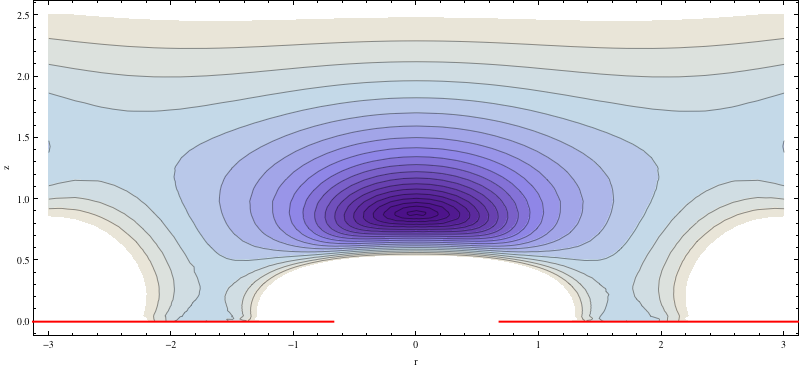## Weighted moorings - to cross or not to cross

The family dinghy was recently relocated to a new spot in the marina, and the question arose: should I cross the back moorings or run them straight?

To add some background, this is a rather small dinghy in a spot suited for a small sailing boat, i.e. a long and narrow spot. This means I have over 2m out to the rear poles, but not much space on the sides. Also, the moorings are weighted, i.e. at each rear pole there is a pulley with a line running to a submerged weight. This ensures that the moorings can just be clicked in and out, which is critical since the point of the dinghy is easy access to water.

This figure shows the geometry: $$C$$ and $$D$$ are the poles, $$F$$ and $$G$$ are the rear corners of the dinghy.

For fixed moorings, there is no doubt that crossing the mooring lines (i.e. using the red lines in the figure) would be the most stiff and least tide sensitive solution.

For weighted moorings, my intuition told me to do the same -- but it did not perform so well, so I went back and did the math. Turns out that not crossing the moorings was more than twice as stiff as crossing.

My first approach was to use geogebra to analyse the moorings, since it is a purely geometrical problem: to find the stiffness, we must compute the variation in the combined mooring length (i.e. $$m+n$$ vs $$i+j$$).

Geogebra actually worked quite well: I could build a parametrized model (see geogebra solution), and using the "locus" tool, it was obvious that not crossing was the way to go.

Still, I could not figure out how to get geogebra to compute the curvature of the locuses (loci?), and decided to have a go with the sympy geometry modlue as well.

This turned out to be a very nice experience. The whole notebook is here, but basically, defining the whole geometry was simply:

a,b,c,d, v = sympy.symbols('a b c d v')
A = geom.Point(-a, 0)
F, G  = [geom.Point(0, dy).rotate(v, pt=A) for dy in (b/2, -b/2)]
C, D = [geom.Point(c, dy) for dy in (d/2, -d/2)]


In six more cells, I get plots of combined mooring line lengths and a symbolic derivation of the stiffness for the two systems:

All in all, I was very impressed with sympy and expect that it will take over the role of geogebra, mathematica etc. in my day to day activities.

## Hello To Hugo

Just prodding the slugs...

Testing mmark with inline ($$x$$), and display math:

$H(X) = \langle I(X) \rangle$

## Piping input to the gcc preprocessor

Spent a while hacking this little pipe to grab the configuration constants for the Marline firmware on my Rostock 3D printer:

echo "$(grep -e '^#define' Configuration.h)$(echo -e "\nDELTA_DIAGONAL_ROD")" | gcc -E - | tail -n 1

The first part,

echo "$(grep -e '^#define' Configuration.h)$(echo -e "\nDELTA_DIAGONAL_ROD")"


will spit out all the #define lines in Configuration.h, followed by a newline (echo -e) and the symbol I am after. Having newlines within bash variables still feels like magic :)

After that, I rely on standard Unix notation to make gcc use standard input (“-”). The gcc documentation doesn’t seem to mention this possibility.

Note to self: find a way of using markdown on Blogger – straight HTML and the “Composer” are equally horrible for geeky stuff.

## Revival

After a long downtime, I’ll try reviving this blog mostly as a place to chronicle my experiences with a newly build 3D printer.

First, let’s get MathJax online. Looking through the docs, it looks like what I need to match the StackEdit/GitHub configuration is the following (at the end of the <head> block):

<script type="text/x-mathjax-config">     MathJax.Hub.Config({         tex2jax: {             inlineMath: [ ['$','$'] ],             displayMath: [ [ '$$', '$$'] ],             processEscapes: true nbsp;    }}); </script> <script src="http://cdn.mathjax.org/mathjax/latest/MathJax.js?config=TeX-AMS_HTML" type="text/javascript">

Below should be a displayed equation
$$E^2 = m^2 + p^2$$
the same could be inlined like so: $E^2 = m^2 + p^2$

Am of course planning on moving it all to a static system like all the cool kids, but not right now…

## Worlds deepest ring trap!

Yup, I am quite sure they will never be deeper than this.Squeezing any harder from the top would send the poor ions flying out the side.